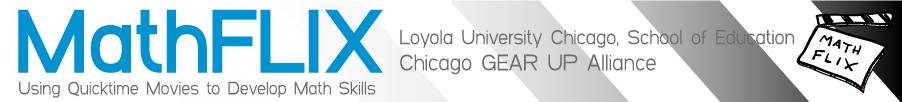### Content Index: ComputerComputer: Database - Computer: Word Processing

• Computer: Database
• survey
• vocabulary
• Computer: Drawing
• angle
• checkerboard problem
• circle vocabulary
• congruent
• diameter
• fraction squares
• fundamental counting principle
• nets
• number line
• palindromes
• prime numbers
• problem solving: Measurement
• problem solving: number and operations
• polyhedra
• quilt patterns
• reflex
• riddles
• scale
• Sieve of Eratosthenes
• similar polygons
• square numbers
• tessellations
• Computer: Graphing
• bar graph
• coordinate geometry
• line graph
• number sequence
• pictograph
• problem solving: data analysis and graphing
• scatter plots
• Computer: History
• Computer: Painting
• cube
• algebra
• area & perimeter
• basics
• coin combinations
• coordinate geometry
• creating
• circumference
• decimals
• fibonacci
• formatting
• fraction
• function
• graphing
• integers
• interest
• multiples
• polygons
• problem solving: algebra
• problem solving: data analysis and probability
• problem solving: measurement
• problem solving: number and operations
• puzzles
• square numbers
• time
• volume
• Computer: Word Processing
• flash cards